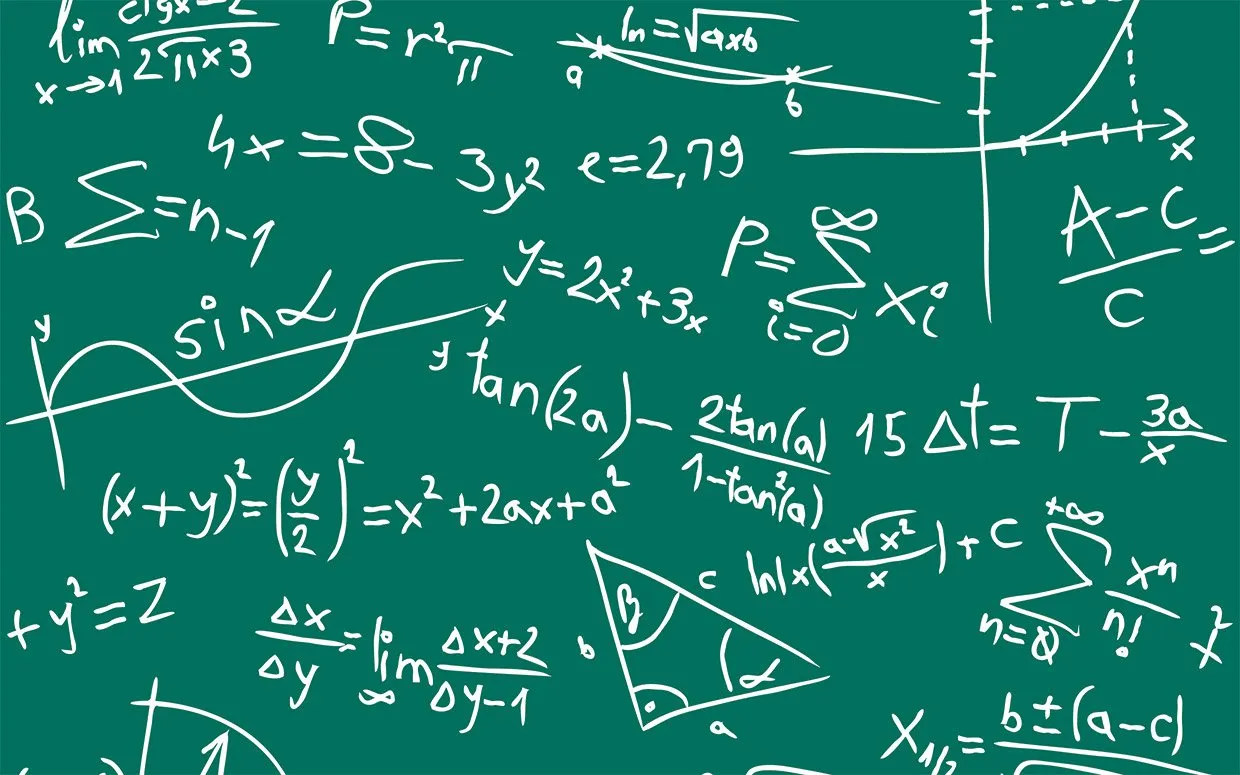# Mathematics - Ordinary first-order Differential equations (part 2)Hey it's me again drifter1! Today we continue with the 2nd part of first-order ODE's from my Mathematics series. There are 6 basic types in total and here we will discuss the 3 remaining. Exercises will come in the next post and will be about all the 1st-order types.

So, let's get straight into it!

### Linear n-order

To understand what a 1st-order linear ODE is, we might first want to get into how n-order ones look and which constraints they have.

A n-order ODE is linear when:

• The function and it's derivatives (y, y', y'', ..., y^(n)) don't come with exponentials/powers
• The coefficients of the function and it's derivatives are a function of x and so f(x) or a number c

You might remember from last time that Homogeneous are converted into Separable ones.

In the same way, the 1st-order linear ODE's are very useful, cause Bernoulli ones get converted into a Linear ODE. Also, Riccati ones get converted into a Bernoulli and then into a Linear.

So, let's get into how we solve 1st-order linear ODE's.

### Solving Linear 1st-order ODE's

A linear 1st-order ODE is of the form:

y' + P(x)*y = Q(x)

There are two main solving methods.

Solution 1:

Multiply both sides of the equation with a new function u = u(x).

We want a u so that: u'y = Puy

Doing some calculations we end up with:

u = e ^ integral[P(x)dx]

and

y = (1/u) * integral[u*Q(x)dx]

That way we can find our solution y.

Solution 2:

We substitute y by setting y(x) = g(x)*Y(x)

We want a g so that: g'/g + P = 0 => Y' = Q/g

By doing some calculations we end up with:

g = e ^[-integral(Pdx)]

and

Y = integral[(Q/g)dx] + c

That way with substitution again we get our y from g and Y.

### Solving Bernoulli ODE's

A Bernoulli ODE is of the form:

y' + P(x)y +Q(x)y^a = 0, where a != 0,1 and real.

We solve u(x) = y(x) ^ (1-a) that will give us a linear ODE.

Solving the linear ODE with the previous method we get u.

From u we can then get our solution y.

### Solving Riccati ODE's

A Riccati ODE is of the form:
y' + P(x)*y^2 + Q(x)*y + R(x) = 0

By substituting y with y = Y + h we end up with a sum of two ODE's:

1. A Bernoulli ODE with Y
2. A Riccati with h

We solve 1 to find Y from the Bernoulli ODE and h is given to us from the beginning as a solution of our ODE, or we can find it easily.

That way by substituting we get our final solution y.

You can see that in general we try to do changes of the form: y =gY + h

### Image sources:### Previous posts of the series:

Introduction -> Definition and Applications

First-order part(1) ->  Separable, homogeneous and exact 1st-order ODE's

And this is it for today and I hope that you enjoyed it!

Next time we will get into exercises for 1st-order ODE's.

Ciao!

H2
H3
H4
3 columns
2 columns
1 column
1 Comment